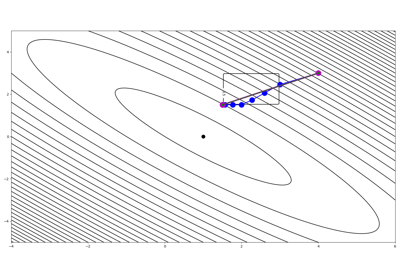# pyproximal.L2#

class pyproximal.L2(Op=None, b=None, q=None, sigma=1.0, alpha=1.0, qgrad=True, niter=10, x0=None, warm=True, densesolver=None, kwargs_solver=None)[source]#

L2 Norm proximal operator.

The Proximal operator of the $$\ell_2$$ norm is defined as: $$f(\mathbf{x}) = \frac{\sigma}{2} ||\mathbf{Op}\mathbf{x} - \mathbf{b}||_2^2$$ and $$f_\alpha(\mathbf{x}) = f(\mathbf{x}) + \alpha \mathbf{q}^T\mathbf{x}$$.

Parameters
Oppylops.LinearOperator, optional

Linear operator

bnumpy.ndarray, optional

Data vector

qnumpy.ndarray, optional

Dot vector

sigmaint, optional

Multiplicative coefficient of L2 norm

alphafloat, optional

Multiplicative coefficient of dot product

qgradbool, optional

Add q term to gradient (True) or not (False)

niterint or func, optional

Number of iterations of iterative scheme used to compute the proximal. This can be a constant number or a function that is called passing a counter which keeps track of how many times the prox method has been invoked before and returns the niter to be used.

x0np.ndarray, optional

Initial vector

warmbool, optional

Warm start (True) or not (False). Uses estimate from previous call of prox method.

densesolverstr, optional

Use numpy, scipy, or factorize when dealing with explicit operators. The former two rely on dense solvers from either library, whilst the last computes a factorization of the matrix to invert and avoids to do so unless the $$\tau$$ or $$\sigma$$ paramets have changed. Choose densesolver=None when using PyLops versions earlier than v1.18.1 or v2.0.0

**kwargs_solverdict, optional

Dictionary containing extra arguments for scipy.sparse.linalg.lsqr solver when using numpy data (or pylops.optimization.solver.lsqr and when using cupy data)

Notes

The L2 proximal operator is defined as:

$prox_{\tau f_\alpha}(\mathbf{x}) = \left(\mathbf{I} + \tau \sigma \mathbf{Op}^T \mathbf{Op} \right)^{-1} \left( \mathbf{x} + \tau \sigma \mathbf{Op}^T \mathbf{b} - \tau \alpha \mathbf{q}\right)$

when both Op and b are provided. This formula shows that the proximal operator requires the solution of an inverse problem. If the operator Op is of kind explicit=True, we can solve this problem directly. On the other hand if Op is of kind explicit=False, an iterative solver is employed. In this case it is possible to provide a warm start via the x0 input parameter.

When only b is provided, Op is assumed to be an Identity operator and the proximal operator reduces to:

$\prox_{\tau f_\alpha}(\mathbf{x}) = \frac{\mathbf{x} + \tau \sigma \mathbf{b} - \tau \alpha \mathbf{q}} {1 + \tau \sigma}$

If b is not provided, the proximal operator reduces to:

$\prox_{\tau f_\alpha}(\mathbf{x}) = \frac{\mathbf{x} - \tau \alpha \mathbf{q}}{1 + \tau \sigma}$

Finally, note that the second term in $$f_\alpha(\mathbf{x})$$ is added because this combined expression appears in several problems where Bregman iterations are used alongside a proximal solver.

Methods

 __init__([Op, b, q, sigma, alpha, qgrad, ...]) affine_addition(v) Affine addition chain(g) Chain grad(x) Compute gradient postcomposition(sigma) Postcomposition precomposition(a, b) Precomposition prox(*args, **kwargs) proxdual(**kwargs)

## Examples using pyproximal.L2#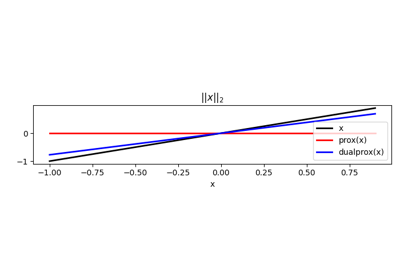Norms

Norms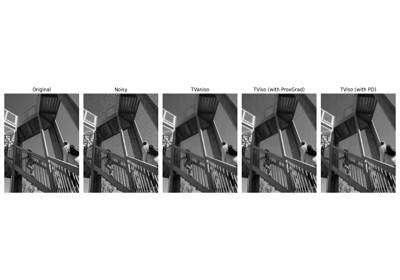Denoising

Denoising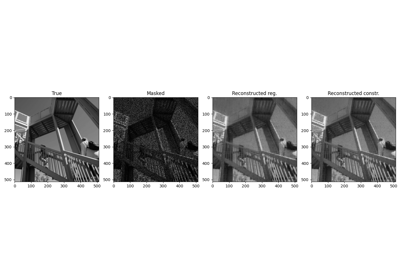Low-Rank completion via SVD

Low-Rank completion via SVD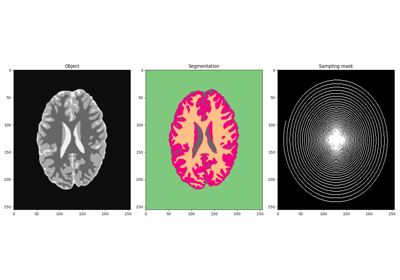MRI Imaging and Segmentation of Brain

MRI Imaging and Segmentation of Brain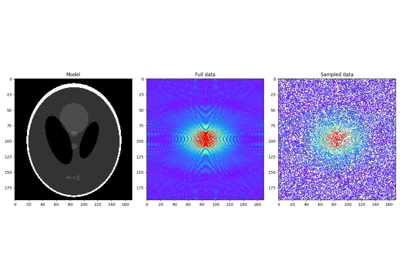Plug and Play Priors

Plug and Play Priors